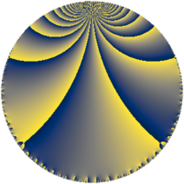# Properties

 Label 207.6.oLevel $207$ Weight $6$ Character orbit 207.o Rep. character $\chi_{207}(5,\cdot)$ Character field $\Q(\zeta_{66})$ Dimension $2360$ Sturm bound $144$

# Related objects

## Defining parameters

 Level: $$N$$ $$=$$ $$207 = 3^{2} \cdot 23$$ Weight: $$k$$ $$=$$ $$6$$ Character orbit: $$[\chi]$$ $$=$$ 207.o (of order $$66$$ and degree $$20$$) Character conductor: $$\operatorname{cond}(\chi)$$ $$=$$ $$207$$ Character field: $$\Q(\zeta_{66})$$ Sturm bound: $$144$$

## Dimensions

The following table gives the dimensions of various subspaces of $$M_{6}(207, [\chi])$$.

Total New Old
Modular forms 2440 2440 0
Cusp forms 2360 2360 0
Eisenstein series 80 80 0

## Trace form

 $$2360 q - 27 q^{2} - 16 q^{3} - 1865 q^{4} - 33 q^{5} + 149 q^{6} - 11 q^{7} - 460 q^{9} + O(q^{10})$$ $$2360 q - 27 q^{2} - 16 q^{3} - 1865 q^{4} - 33 q^{5} + 149 q^{6} - 11 q^{7} - 460 q^{9} - 44 q^{10} - 33 q^{11} - 1354 q^{12} - 9 q^{13} - 33 q^{14} + 9240 q^{15} + 28599 q^{16} + 11499 q^{18} - 44 q^{19} - 33 q^{20} - 12529 q^{21} - 7839 q^{23} + 37324 q^{24} + 72047 q^{25} + 17921 q^{27} - 44 q^{28} - 33345 q^{29} + 33594 q^{30} - 4443 q^{31} - 26619 q^{32} + 82500 q^{33} - 11 q^{34} - 7465 q^{36} - 44 q^{37} - 33 q^{38} - 45232 q^{39} + 51139 q^{40} + 72153 q^{41} - 22 q^{42} - 11 q^{43} - 45876 q^{46} - 6888 q^{47} - 8420 q^{48} - 238045 q^{49} + 37977 q^{50} - 22 q^{51} - 27110 q^{52} + 51300 q^{54} - 40124 q^{55} - 63393 q^{56} - 92455 q^{57} + 3368 q^{58} + 167607 q^{59} - 420662 q^{60} - 11 q^{61} - 7227 q^{63} + 792482 q^{64} + 589248 q^{65} + 312345 q^{66} - 11 q^{67} + 75567 q^{69} + 27002 q^{70} - 433396 q^{72} - 36 q^{73} - 322113 q^{74} - 596533 q^{75} - 11 q^{76} - 90501 q^{77} - 745617 q^{78} - 11 q^{79} - 1851896 q^{81} - 151126 q^{82} + 147378 q^{83} + 978472 q^{84} + 6241 q^{85} - 33 q^{86} - 285220 q^{87} - 22539 q^{88} + 3273688 q^{90} - 118035 q^{92} - 146696 q^{93} - 4476 q^{94} - 129837 q^{95} - 951454 q^{96} + 291412 q^{97} - 1349326 q^{99} + O(q^{100})$$

## Decomposition of $$S_{6}^{\mathrm{new}}(207, [\chi])$$ into newform subspaces

The newforms in this space have not yet been added to the LMFDB.